Russian Journal of Earth Sciences
Vol 1, No. 1, July 1998
Translated December 1998

# On the effects of the inertia ellipsoid triaxiality in the theory of nutation

S. M. Molodensky

Joint Institute of the Physics of the Earth, 10 Bolshaja Gruzinskaja, Moscow 123810

### Abstract

Modern nutation theories of the Earth and planets use the assumption, that the planet’s inertia ellipsoid is symmetrical about the axes of rotation. Below we estimate the effects of the inertia ellipsoid triaxiality accurate to ((B-A)/(C-A))4, where A, B, C are equatorial and polar principal moments of inertia, respectively.

### 1. Introduction

As known, the nutational motion of the Earth and planets in space is fully determined by Euler angles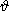E, fE, yE, which describe the position of the body-fixed (Tesserand's) axes ( x, y, z ) with respect to an immobile reference frame. At the same time, dynamic equations of motion of an actual planet model define only the components (wx, wy, wz) of the angular velocity vector w with respect to a moving reference frame. In the case of an axially symmetrical planet (equatorial moments of inertia satisfy the condition A = B ) the Euler angles are connected with these components through well-known Poinsot's formulae (see, for example, [Landau and Lifshitz, 1964]), but in the case A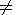B Euler's relations betweenE, fE, yE and (wx, wy, wz) present a rather complicated (nonlinear) system of ordinary differential equations which has not been investigated in all details as yet.

As was shown by Zharkov et al. , in the case of small violation of the axial symmetry (if the parameter

h = (A-B)/(C-A)

satisfies the condition |h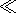1| ) Euler's equations can be solved by using the perturbation method in powers of this parameter; in such an approach, first-order (in h ) corrections describe only short-period (semidiurnal) perturbations of the valuesE, fE, yE. Nevertheless, this result cannot be considered as a final solution of the problem under consideration, because second-order corrections of the order of h2 can contain also long-period terms, which perturb not only instant values of the nutational amplitudes, but also their mean values. Modern VLBI measurements are most sensible just to these terms, and their analysis is most interesting for any practical purposes. Below we present a simple analytical expression that describes these corrections with the accuracy of the order of h4.

### 2. Statement of the Problem

As mentioned above, modern astrometric measurements determine the motion of fixed points of a planetary surface R with respect to an immobile reference frame usually connected with extragalactic objects. At the same time, well-known Liouville's equation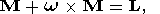(1)

(where w is the angular velocity of the Tesserand's reference frame (x, y, z), M is the angular momentum of the planet, L is the torque of external forces, and the dot above symbol denotes the time derivative) determines only the motion of the vector with respect to the moving system of coordinates (x, y, z). To express the components of the vector R in terms of the known values (wx, wy, wz), it is convenient to use the well-known Poinsot's kinematic relations (see, for example, [Zharkov et al., 1996]) which connect the trajectory of the vector w with respect to the moving reference frame (x, y, z) (polhode) and the trajectory of the same vector in space (herpolhode).

In the case of an axially symmetrical rigid planet (A=B‚ and the products of inertia Iik vanish), the components M and w are connected by the well-known relation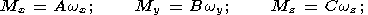(2)

and the torque L may be represented in the form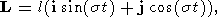(3)

where i, j are unit vectors oriented along the axes x and y, respectively,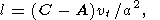(4)

vt is the amplitude of the nearly diurnal tidal potential,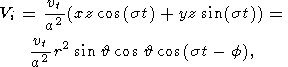(5)

is the mean radius of the planet, (r,, f ) are spherical coordinates, and s is tidal frequency.

In the most general case, Liouville equation (1) represents a system of three nonlinear ordinary differential equations with respect to three unknown functions wx(t), wy(t), wz(t). In the case h = 0, it has a very simple exact solution. In fact, substituting (2)-(4) into (1), we obtain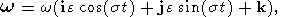(6)

where k is a unit vector that coincides with the z axis and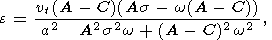(7)

is the amplitude of nutational motion with respect to the moving reference frame (x, y, z).

The fact that the amplitudes the x and y components of vector w in (6) are equivalent is a direct consequence of the axial symmetry of the problem under consideration. In view of this, the surfaces of polhode and herpolhode are exactly conical; in accordance with Poinsot's kinematic relations, the ratio of apex angles of these cones is defined by the relation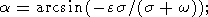(8)

the amplitude of nutational motion of a vector R in the body-fixed reference frame (x, y, z) is equal to a + e in the case of prograde nutational motion (if the directions of the w motion and nutation coincide) and to in the opposite case of retrograde motion.

At h = 0 the solutions of dynamic and kinematic nonlinear equations can be rather complicated. Because long-period perturbations are most important in practical applications, below we use the method of its approximate solution based on the following procedure:

1. Dynamic equations (1) provide expressions for the components wx(t), wy(t), wz(t) of the vector w with respect to the moving reference frame, in which the effects of the inertia ellipsoid triaxiality are taken into account;

2. Inverting Euler's kinematic relations between the known components wx(t), wy(t), wz(t) of the vector w in the moving reference frame, the components w1(t), w2(t), w3(t) of the same vector in space, and Euler's anglesE, fE, yE, these angles can be expressed in terms of wx(t), wy(t), wz(t). Taking into account, that the kinematic relations are nonlinear, we use the first-order method of perturbations with respect to the small parameter h.

3. To connect short-period first-order perturbations of the functions w1(t), w2(t), w3(t) and long-period second-order perturbations of the same functions, we use the well-known Poinsot's theorem stating that the rotation of an arbitrary triaxial rigid body may be represented as the rolling of the polhode over the herpolhode without sliding. In accordance with this theorem, the ratio of trajectory lengths of the vector w in the moving and fixed reference frames L1 and L2 is equal to the ratio of the corresponding periods. The perturbation of the trajectory L2 in turn consists of (1) the known terms which describe semidiurnal oscillations and (2) unknown terms of higher orders of smallness which describe the mean radius of this trajectory. As is shown below, this permits the mean radius of the trajectory L2 to be found with sufficient accuracy (of the order of h4 ).

### 3. Tidal Torque for a Triaxial Body

Using expression (5) for the components of tidal potential in Cartesian coordinates, it is easy to find the tidal torques which act on an axially nonsymmetrical planet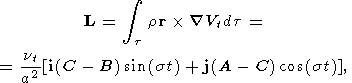(9)

where t is the total volume of the Earth. Replacing the relation between wy and My in equations (2) by the formula My = Bwy and then substituting (2) and (9) in Liouville's equation (1), we obtain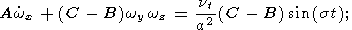(10a)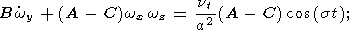(10b)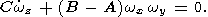(10c)

Using relations (6) and (7), it is easy to see that the ratio of terms Cwz and ( B-A)wxwy in (10c) is of the order of hevt /(ga), where e is the flattening of the planet and g is the gravity on its surface. This ratio is negligibly small for all planets of the solar system, and the approximation wz = w = const is valid with a very high accuracy. In this approximation, the solution of equations (10a) and (10b) describing forced nutation and precession is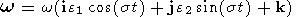(11a)

where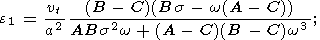(11b)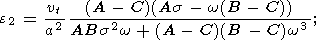(11c)

Substituting relations (11) in the well-known Euler relations between Euler angles (E, fE, yE ) and ( wx, wy, wz ) [Landau and Lifshitz, 1964]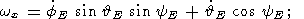(12a)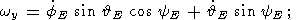(12b)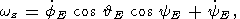(12c)

we obtain a system of three nonlinear ordinary differential equations with respect to three unknown functionsE, fE, yE. In the case of an axially symmetrical body ( A=B and e1 = e2 = e, where e is defined by (7)) these equations have an elementary (Poinsot) solution in the form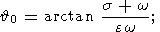(13a)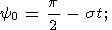(13b)

and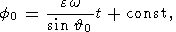(13c)

which show, that the angular frequency and amplitude of nutational motion in space are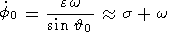and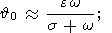(13d)

(if this amplitude satisfies the condition |0|1 )

### 4. First-order Approximation for a Slightly Triaxial Body

To estimate the effects of triaxiality of the planet in the first approximation, we first perform some simple transformations. Multiplying (12a) and (12b) by cosyE and sinyE and then subtracting the results, we expressE in terms of e1, e2 as follows: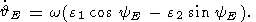(14a)

Analogously, multiplying (12a) and (12b) by sinyE and cosyE and then summing the results, we have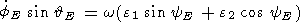(14b)

To linearize these equations, their solutions are represented by the superposition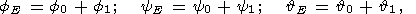(15)

where f0, y0,0 are the solutions of axially symmetrical problem (13a)-(13c), and f1, y1,1 are small corrections due to the triaxiality effects of the inertia ellipsoid.

Substituting (15) and (11a) into (14a), (14b), and (12c) and neglecting the terms of the order of h2, we obtain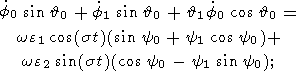(16a)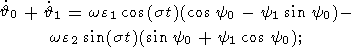(16b)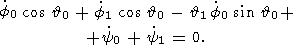(16c)

Transforming, in accordance with (13b), the main terms in the right-hand sides of these equations,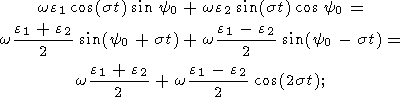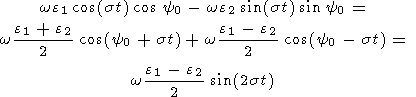and taking into account, that the zero-order values of f0,0, y0 are connected with e, w and s by relations (13), we obtain, after differentiation of these relations with respect to the time,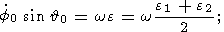(17a)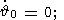(17b)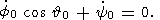(17c)

Using these relations, equations (16) can be rewritten as follows: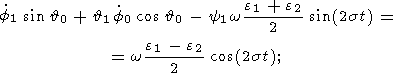(18a)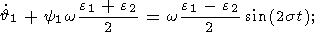(18b)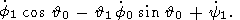(18c)

Eliminating0 and f0 from these relations with the help of (13a) and (17c), we finally obtain the system of three inhomogeneous linear ordinary differential equations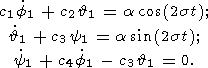(19)

with the coefficients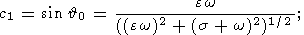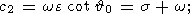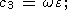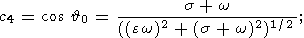and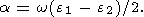Since the system of equations (19) is of the third order, it has three linearly independent homogeneous solutions. It is easy to show, however, that these solutions are not interesting for the problem under consideration, because they describe the small variations in Euler angles caused by an arbitrary infinitesimal rotation of the fixed reference frame.

The inhomogeneous solution of (19) has the form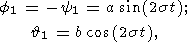(20)

where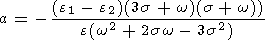and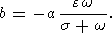These expressions fully determine first-order short-period perturbations of the nutational and precessional motion caused by the triaxiality of the planet’s figure.

To find short-period motion perturbations of the vector w, we introduce a fixed Cartesian reference frame (  e1, e2, e3 ) in such a way that the axis e3 coincides with the axis of the unperturbed cone of herpolhode, and fE is equal to the angle between the nodal line and vector e1. Then the relations between components ( w1, w2, w3 ) and Euler angles (E, fE, yE ) are completely analogous to (12a)-(12c) after replacement of fE by yE and yE by fE :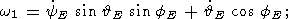(21a)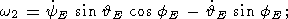(21b)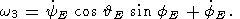(21c)

In the case of nutational motion of any actual planet, its spatial amplitude ew / (s + w) does not exceed a few seconds of arc, and we can use, instead of (13a), a more simple expression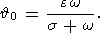(22)

Substituting (15), (13b), (13c), (22), and (17) into (21), we have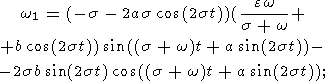(23a)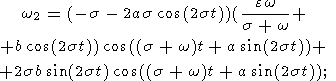(23b)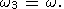(23c)

Like the first-order corrections to Euler angles (20), these expressions contain only short-period (semidiurnal) perturbations.

### 5. Long-period terms in the second-order approximation

Now, we will use the well-known Poinsot's theorem stating that the rolling of polhode over herpolhode takes place without sliding. On the strength of this theorem, a general method for the calculation of long-period herpolhode perturbations may be formulated as follows. Let the total length of the vector w trajectory in the moving reference frame ( x, y, z ) is defined by the expression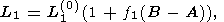(24a)

where L1(0) is the length of the vector in the case of an axially symmetrical planet ( A=B ) and f1(B-A) is a small correction due to +effects of triaxiality. Because this trajectory coincides with circle (6) if A=B and with ellipse (11a) if AB, the function f1 actually describes the known difference between the length of ellipse (11a) with the semiaxes we1, we2 and the length 2pwe of circle (6).

Likewise, using relations (23), the total length of the w trajectory in the fixed reference frame (  e1, e2, e3 ) can be represented in the form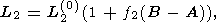(24b)

where L2(0) = |s / (s + w)| L1(0) is the total length of the herpolhode in the biaxial case and f2(B-A) describes the difference between the lengths in the triaxial and biaxial cases.

As mentioned before, the absence of exact expressions for the Euler angles in terms of known components wx(t), wy(t) precludes direct determination of this function. However, expressions (23) readily provide the part of this function f2(s)(B-A) which is connected with short-period (semidiurnal) perturbations of herpolhode. Using this value, the total perturbation of herpolhode length can be represented as a sum of the known short-period perturbation L2(0)f2(s)(B-A) and unknown long-period perturbation L2(0)f2(1)(B-A) that describes variation in the "mean'' herpolhode radius: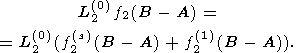(25a)

In accordance with Poinsot's theorem, the condition of the polhode rolling over herpolhode without sliding is that the trajectories described by the angular velocity vector during equal time intervals have the same lengths in moving and fixed reference frames: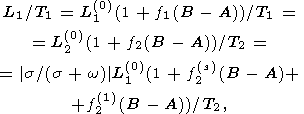where T1 and T2 are full revolution periods of the vector w in the moving and fixed reference frames, respectively. Since these values are connected through the relation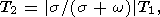the unknown value f2(1)(B-A) can be expressed in terms of the known functions f1(B-A) and f2(s)(B-A) in the following, very simple form: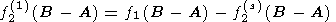(25b)

Then, variation in the mean radius of herpolhode (which coincides with variation in the nutation amplitude dA multiplied by w ) may be represented in the form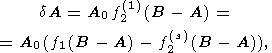(26)

where A0 is the unperturbed nutation amplitude corresponding to the axially symmetrical approximation. In accordance with (11), the complete trajectory length of the vector w in the moving reference frame ( x, y, z ) is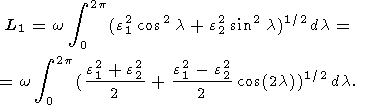Developing the integrand function as the Taylor’s series in small parameter k = (e12 - e22)/(e12 + e22) with the help of (11b) and (11c) and neglecting terms of the order of k4, we have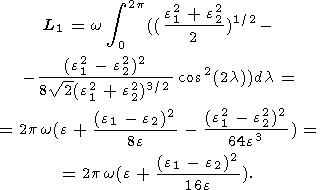(27a)

Comparing this expression and relation (24a) yields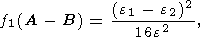(27b)

where the nutation amplitudes e1, e2 and e are defined by expressions (11b), (11c), and (7), respectively.

Strictly speaking, the definition of the complete trajectory length of the vector w in the fixed reference frame (  e1, e2, e3 needs some refinement, because this trajectory is generally nonclosed. In fact, it is obvious that, since the period of short-period perturbations is equal to p/s and the period of nutational motion is equal to 2p/|s + w|, this trajectory is closed only if s = k(s + w), where k is any integer number. Of course, nutation frequencies of actual planets do not satisfy this condition, and the herpolhode is not a closed curve.

In order to apply Poinsot's theorem to the case of nonclosed trajectory of the angular velocity vector in space, we define the "mean length'' of this trajectory as follows: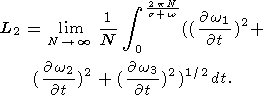(28)

Substituting (23) into (28) and expanding, as before, the integrand function in Taylor's series with the accuracy of the order of k4, we obtain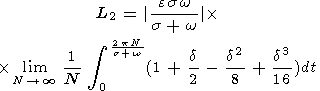(29a)

where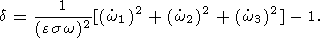(29b)

Substituting (20a-c) into (26a, b), we have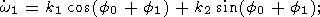(30a)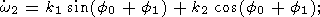(30b)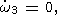(30c)

where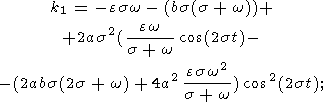(31a)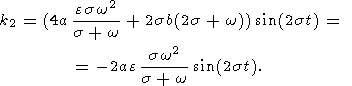(31b)

Substituting these expressions into (29), (30a), and (30b) and neglecting the terms of the fourth and higher orders, we have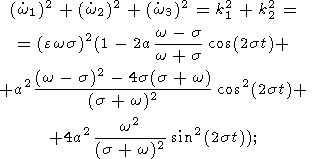(32a)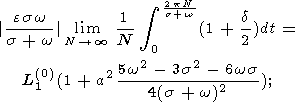(32b)

)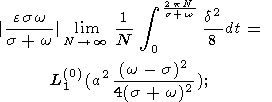(32c)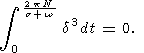(33)

Summing these expressions, we finally obtain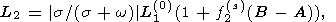(34a)

where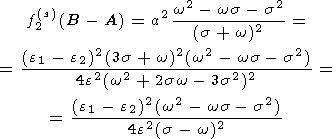(34b)

Substituting (34b) and (26b) into (23), we obtain the final value of the correction to the mean nutation amplitudes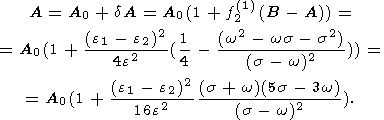(35)

Note that this correction vanishes if s = -w or s = 3w/5. The first root corresponds to the precession frequency. Vanishing of this expression for the precession frequency implies that the precessional constant depends only on the value ‘ - (A+B)/2 but not on the difference of equatorial moments of inertia B-A.

The second root, as well as the denominator root s = -w, corresponds to the case of high nutation frequencies in space as compared with the period of diurnal rotation (these frequencies correspond to the spatial periods of nutational motion Ts = 5T0/8 and Ts = T0/2,

respectively, where T0 = 2p/w is one sidereal day). Nutational motion with the period Ts = T0/2 takes place only in the case of synchronised diurnal and orbital rotation (if at the points of equinoxes the orientation of the planet's principal equatorial moments of inertia is invariable in space).

In this case, the nutation amplitudes are obviously determined not by the mean values of A, B averaged over the total period, but by their "effective'' values in the vicinity of points at which the tidal torque is maximum. In this specific case, the corrections to the mean nutation amplitudes are proportional to (e1 e2)/e rather than (e1 e2)2/e2. This qualitative consideration explains, why second-order correction (35) tends to infinity in the limiting case sw.

#### Acknowledgment

This work was supported by the Russian Foundation for Basic Research, project No. 98-05-64419.

### References

Landau, L. D. and E. M. Lifshitz, Mechanics, Moscow, Nauka, 1964 (in Russian).

Molodensky, S. M., Tides, Nutation, and Internal Structure of the Earth, Moscow, Nauka, 1980, 214 pp. (in Russian).

Zharkov V. N., S. M. Molodensky, E. Groten, A. Brzezinski, and P. Varga. The Earth and its rotation. Low-frequency geodynamics, Heidelberg, Wichman Verlag, 1996, 531 pp.

Load files for print and local use.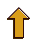This document was generated by TeXWeb (Win32, v.1.0) on February 26, 1999.